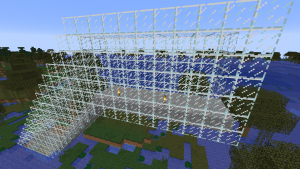# Python in Minecraft 7 – define tunnel route in function

In the previous example the tunnel was horizontal so the value of y for the tunnel was always constant. To make the tunnel route more complicated without having duplicate code we define a function called tunnelvertical(eastposition). For any value of the east position (x value) this function will return the vertical position of the tunnel.

```def tunnelvertical(eastposition):
y = abs(100 - (eastposition - XMIN))
if y < 10:
return 10
if y > 72:
return 72
return y
```

XMIN is a constant we have previously defined in the code showing where the tunnel starts. The shape of this tunnel is horizontal at ground level, then slopes down until it reaches level 10, goes horizontal for a while, then slopes up until it reaches ground level again and finishes off horizontal.

Here is the full code

```import mcpi.minecraft as minecraft
import mcpi.block as block
mc=minecraft.Minecraft.create()
XMIN = -400 # x value at one end of tunnel.
XMAX = -200 # x value at other end of tunnel. Must be greater than XMIN
GROUND = 72 # y position of tunnel at each end
FLOOR = 10  # minimum value of y at bottom of tunnel
ZPOS = -222 # z position for full length of tunnel
TAIL = 10   # length of horizontal at each end of tunnel

# constants for tunnelvertical function
kx = (XMAX + XMIN) / 2
ky = GROUND + TAIL - (XMAX - XMIN) / 2

# tunnelvertical returns a vertical position of the tunnel
# for each value of the x position (eastposition)
def tunnelvertical(eastposition):
y = abs(eastposition - kx) + ky
if y < FLOOR:
return FLOOR
if y > GROUND:
return GROUND
return y

# set the z coordinate for the tunnel which doesn't change for the full length
z = ZPOS

# Initial loop to create a route made of solid glass with a stone base
for x in range(XMIN,XMAX+1):
y = tunnelvertical(x)
mc.setBlocks(x,y,z-2,x,y+6,z+2,block.GLASS)
mc.setBlocks(x,y,z-1,x,y,z+1,block.STONE)

# Second loop to convert solid glass into a tunnel
for x in range(XMIN+1,XMAX):
y = tunnelvertical(x)
# replace centre glass with air to make it a tunnel
mc.setBlocks(x,y+1,z-1,x,y+5,z+1,block.AIR)
# place a torch every 4 positions to light the tunnel
if x % 4 == 0:
mc.setBlock(x,y+1,z+1,block.TORCH.id,5)
```Categories: CoderDojo